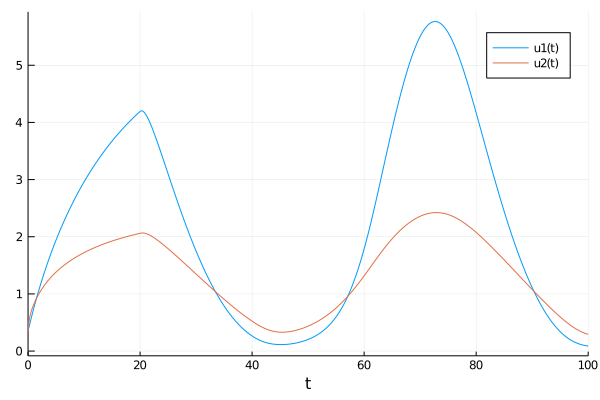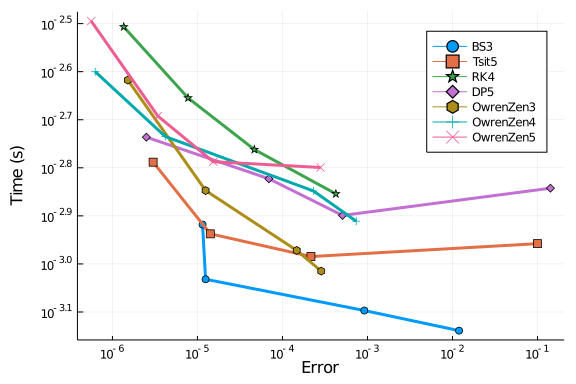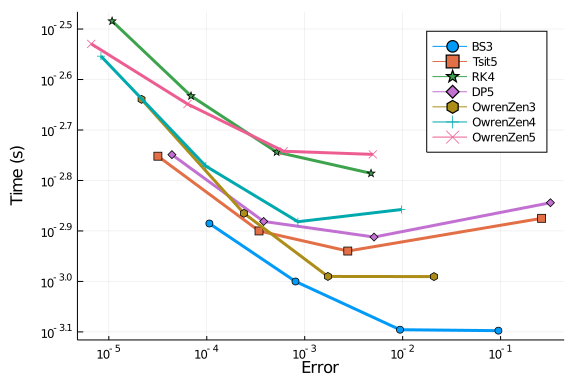# Wheldon, Kirk, and Finlay

We study algorithms for solving constant delay differential equations with a test problem from W.H. Enright and H. Hayashi, "The evaluation of numerical software for delay differential equations", 1997. It is a model of chronic granulocytic leukemia that was published by T. Wheldon, J. Kirk and H. Finlay in "Cyclical granulopoiesis in chronic granulocytic leukemia: A simulation study", 1974, and is given by

\begin{align} y_1'(t) &= \frac{1.1}{1 + \sqrt{10}y_1(t-20)^{5/4}} - \frac{10y_1(t)}{1 + 40y_2(t)} \\ y_2'(t) &= \frac{100y_1(t)}{1 + 40y_2(t)} - 2.43y_2(t) \end{align}

using DelayDiffEq, DiffEqDevTools, DiffEqProblemLibrary, Plots
using DiffEqProblemLibrary.DDEProblemLibrary: importddeproblems; importddeproblems()
import DiffEqProblemLibrary.DDEProblemLibrary: prob_dde_wheldon
gr()

sol = solve(prob_dde_wheldon, MethodOfSteps(Vern9(); fpsolve = NLFunctional(; max_iter = 1000)); reltol=1e-14, abstol=1e-14)
test_sol = TestSolution(sol)
plot(sol)## Low order RK methods

### High tolerances

First we compare final errors of solutions with low order RK methods at high tolerances.

abstols = 1.0 ./ 10.0 .^ (4:7)
reltols = 1.0 ./ 10.0 .^ (1:4)

setups = [Dict(:alg=>MethodOfSteps(BS3())),
Dict(:alg=>MethodOfSteps(Tsit5())),
Dict(:alg=>MethodOfSteps(RK4())),
Dict(:alg=>MethodOfSteps(DP5())),
Dict(:alg=>MethodOfSteps(OwrenZen3())),
Dict(:alg=>MethodOfSteps(OwrenZen4())),
Dict(:alg=>MethodOfSteps(OwrenZen5()))]
names = ["BS3", "Tsit5", "RK4", "DP5", "OwrenZen3", "OwrenZen4", "OwrenZen5"]
wp = WorkPrecisionSet(prob_dde_wheldon,abstols,reltols,setups;names=names,
appxsol=test_sol,maxiters=Int(1e5),error_estimate=:final)
plot(wp)Next we test interpolation errors:

abstols = 1.0 ./ 10.0 .^ (4:7)
reltols = 1.0 ./ 10.0 .^ (1:4)

setups = [Dict(:alg=>MethodOfSteps(BS3())),
Dict(:alg=>MethodOfSteps(Tsit5())),
Dict(:alg=>MethodOfSteps(RK4())),
Dict(:alg=>MethodOfSteps(DP5())),
Dict(:alg=>MethodOfSteps(OwrenZen3())),
Dict(:alg=>MethodOfSteps(OwrenZen4())),
Dict(:alg=>MethodOfSteps(OwrenZen5()))]
names = ["BS3", "Tsit5", "RK4", "DP5", "OwrenZen3", "OwrenZen4", "OwrenZen5"]
wp = WorkPrecisionSet(prob_dde_wheldon,abstols,reltols,setups;names=names,
appxsol=test_sol,maxiters=Int(1e5),error_estimate=:L2)
plot(wp)Both interpolation tests and tests of final error show similar results. BS3 does quite well but only OwrenZen4, OwrenZen5, and RK4 achieve interpolation errors of about 1e-5.

### Low tolerances

We repeat our tests at low tolerances.

abstols = 1.0 ./ 10.0 .^ (8:11)
reltols = 1.0 ./ 10.0 .^ (5:8)

setups = [Dict(:alg=>MethodOfSteps(BS3())),
Dict(:alg=>MethodOfSteps(Tsit5())),
Dict(:alg=>MethodOfSteps(RK4())),
Dict(:alg=>MethodOfSteps(DP5())),
Dict(:alg=>MethodOfSteps(OwrenZen3())),
Dict(:alg=>MethodOfSteps(OwrenZen4())),
Dict(:alg=>MethodOfSteps(OwrenZen5()))]
names = ["BS3", "Tsit5", "RK4", "DP5", "OwrenZen3", "OwrenZen4", "OwrenZen5"]
wp = WorkPrecisionSet(prob_dde_wheldon,abstols,reltols,setups;names=names,
appxsol=test_sol,maxiters=Int(1e5),error_estimate=:final)
plot(wp)